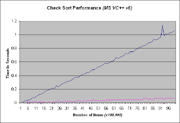580 Pages

## Table of Performance Values

#### Regression Analysis

Console output - (constraints)

```RESULTS: (Check sort)
1.) Total number of items sorted:...................... 1542936
2.) Total primary array size to accommodate the sort:... 10000000
3.) Total secondary array size to accommodate the sort:. 1542936
4.) Total length of key:............................... 8 decimal digits.
5.) Total time for setup per 100,000 items:............ 1.109 seconds.
6.) Total time for sort per 100,000 items:............. 0.078 seconds.
7.) Total time for program:............................ 59.14 seconds.
Press any key to continue
```

#### Class Determination

Class notated sort routines are categorized according to the Chi Square analysis of linear (L), parabolic {P), logarithmic (Ln) and exponential (E) best fit regression analysis applied to their performance data produced under a specified set of constraints. Class notation is used in preference to Theta notation, a form of Big O notation, since the advantage Class notation offers is to provide the values of the equation parameters obtained from regression analysis of the performance data such as in the case of a linear equation best Chi Square fit of the value for slope (or seconds per item) in the case of a Class L (linear equation) category best fit. This additional information allows the programmer or user to select the best sort routine for his own application based upon the actual number of items which must be sorted per unit time or the time limit to sort a specific number of items. Since the Check sort performance best fits the Class L category the values for slope m and constant c for the linear equation f(x)= m*x+c can be determined by a best fit linear regression analysis and provided in the table below:"Blue is setup time, Magenta is sort time."
Class L
Routine Slope c
Setup 0.011481868 0
Sort 0.000677576 0

This method of representation allows performance to be projected for any number of items or time limit. A projection of sort routine performance for any machine can be made using the time required to initialize an array by calculating the ratio of sort time to setup time (which in this case isor 0.0597) or a percentage value of 5.97%. Thus on a different machine providing a setup time slope value of 0.025 the projected sort time would be .025 * 0.0597 or 0.00147531 seconds per item or the ability to sort 67,782,364 items per second assuming the software methods for performing the setup and the sort are the same for each machine.

Community content is available under CC-BY-SA unless otherwise noted.